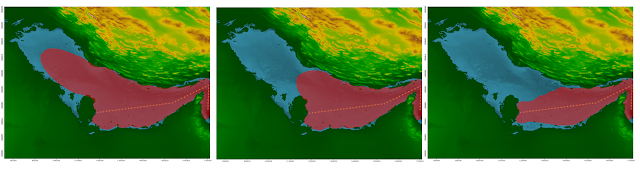## What difference rocks make

Sometimes I get asked how important environmental parameters are when predicting underwater noise levels. I will try to give you a small example of what happens if the sediment is not right in the model.

By the bad fortune of an unlucky intern the entire Seabed of the Persian Gulf was turned into rock! Well, if the sound if mostly carried by the water how much of a difference can it make!?Figure 1. Three scenarios: rocky seabed, mixed seabed and sandy seabed. Red area indicate levels over 110 dB.

The hardness of the rock means that the speed of sound in it is much higher than in the water. Snell's Law tells of about refraction and reflection between two substances with different soundspeed.Figure 2. Snell's Law

In sand the soundspeed is roughly 1700 m/s, in basalt (what I used above) it's about 5300 m/s and in water I'll use the familiar 1500 m/s.

I will use the first part of Snell's law for this (sin(θ1)/sin(θ2)=v1/v2)
A little fiddling around shows that in the rock situation, if the angle of incident for the sound is more than 16.5 degrees (or 18 %)  from vertical, the sound is reflected by the sediment rock rather than propagating into it (within this "window" ~80 % of the sound is reflected anyway due to impedance differences, see Fig 3.).

This efficiently keeps the sound in a channel formed by the boundaries of the seabed and the water surface, and only lets a minimal amount of energy leak out of it.

For the sandy seabed the corresponding angle is 62 degrees from vertical, meaning that 70 % of incidence angles result in sound transmitted into the sediment (with about 40 % being reflected due to impedance differences)

The below equation can be used to estimate reflection between materials of different impedances.Figure3. Reflection coefficients can be calculated from this equation. θ is angle from 90 degrees to plane, ρ is the density and c soundspeed, i & t are incident & transmitted.

The sandy seabed is much more "leaky", and much of the energy propagates into it, and dissipates there, leaving the surroundings quieter.

So seabed is important.

In the center image of Figure 1 (up top), I have placed some rock in the center of the Persian Gulf. In the figure below you can see that there are some dots, these are my fictitious measuring points. All the red points indicate a rock seabed, yellow ones a sandy one.Figure 4. Red dots are areas of rock (in this example) while yellow dots represent sand. Areas between points are interpolated from a combination of its surrounding points.

Apart from being an example of what a mixed bathymetry can do to modelling results, This option of having different bathymetry at different locations is one of the features in the new dBSea 2.0 - coming out at the end of this month. We are quite excited, and hope you are too.Figure 5. Animation of exclusion zone with sandy seabed.

As a visualisation feature, we've added animation export for moving sources :)